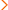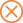Home > ed > PSAT Math - An Overview of the PSAT Math Sections

# PSAT Math - An Overview of the PSAT Math Sections

The PSAT Math section is composed of two sub-sections: a 45-minute calculator-optional section, and a 25-minute no-calculator section. These sections include a total of 48 questions: 40 multiple-choice questions and 8 student-produced response (or "grid-in") questions. The multiple-choice questions provide four possible answers; students must determine which of the four choices is the correct solution to the question. The grid-in questions require students to calculate and grid the correct answers; no answer choices are given. Some grid-in questions can have more than one correct answer.

The PSAT Math questions focus on four areas: heart of algebra; problem solving and data analysis; passport to advanced math, and additional topics in math, including limited geometry, trigonometry, and pre-calculus. The chart below provides more information about the specific skills these questions test. While students are allowed to use a calculator on a portion of the PSAT math section, all test-takers should check the College Board website for details about approved and restricted calculators.

Math Area Examples
Heart of Algebra

analyzing and fluently solving equations and systems of equations; creating expressions, equations, and inequalities to represent relationships between quantities and to solve problems; rearranging and interpreting formulas

Problem Solving & Data Analysis

substitution and simplifying algebraic expressions; properties of exponents; algebraic word problems; linear equations and inequalities; systems of equations and inequalities; rational and radical equations; equations of lines; absolute value; direct and inverse variation; quadratic equations; algebraic functions

area and perimeter of a polygon; area and circumference of a circle; volume; Pythagorean theorem; isosceles, equilateral, and right triangles; parallel and perpendicular lines; coordinate geometry; slope; transformations

data interpretation; statistics; mean, median, and mode; probability

To access sample PSAT Math multiple-choice questions, visit the College Board website.

To access sample PSAT Math grid-in questions, visit the College Board website.

Related Topics

LEARN MORE